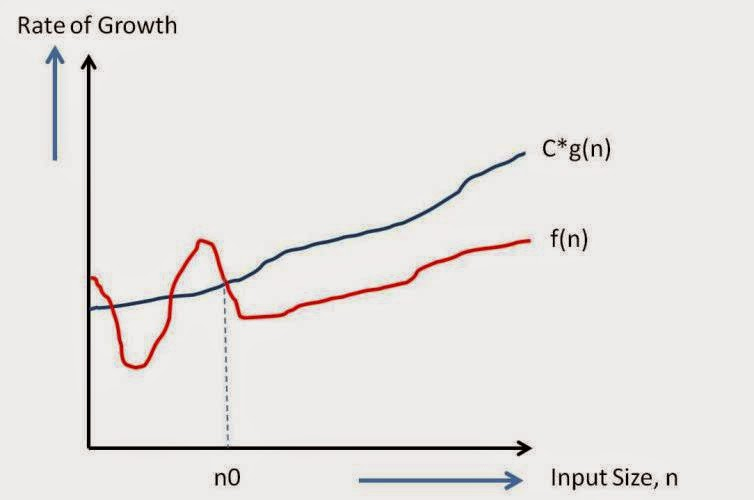# How will you compare algorithms?

You can compare algorithms by its rate of growth with input size n

Lets take a example.For solving same problem, you have two functions:
f(n) =4n^2 +2n+4 and f(n) =4n+4

For f(n) =4n^22 +2n+4
so here
f(1)=4+2+4
f(2)=16+4+4
f(3)=36+6+4
f(4)=64+8+4
….
As you can see here contribution of n^22 increasing with increasing value of n.So for very large value of n,contribution of n^2 will be 99% of value on f(n).So here we can ignore low order terms as they are relatively insignificant as described above.In this f(n),we can ignore 2n and 4.so
n^2+2n+4 ——–>n^2

For f(n) =4n+4
so here
f(1)=4+4
f(2)=8+4
f(3)=12+4
f(4)=16+4
….
As you can see here contribution of n increasing with increasing value of n.So for very large value of n,contribution of n will be 99% of value on f(n).So here we can ignore low order terms as they are relatively insignificant.In this f(n),we can ignore 4 and also 4 as constant multiplier as seen above so
4n+4 ——–>nSo here n is highest rate of growth.
Point to be noted :
We are dropping all the terms which are growing slowly and keep one which grows fastest.

### Big O Notation:

This notation is used for theoretical measure of execution of an algorithm. It gives tight upper bound of a given function. Generally it is represented as f(n)=O(g(n)) and it reads as “f of n is big o of g of n”.

Formal definition:

f(n) = O(g(n)) means there are positive constants c and n0, such that 0 ≤ f(n) ≤ cg(n) for all n ≥ n0. The values of c and n0 must not be depend on n.When you say O(g(n)) , It means it will never be worst than g(n). Having said that it means O(g(n)) includes smaller or same order of growth as g(n).

So O(n) includes O(n),O(logn) and O(1).

So O(g(n)) is a good way to show complexity of algorithm.

Lets take some example and calculate value for c and n0.
1. f(n)=4n+3
Writing in a form of f(n)<=c*g(n) with f(n)=4n+3 and g(n)=5n

4n+3<=5n for n0=3 and c=5.

or 4n+3<=6n for n0=2 and c=6
Writing in a form of f(n)<=c*g(n) with f(n)=4n+3 and g(n)=6n
so there can be multiple values for n0 and c for which f(n)<=c g(n) will get satisfied.

2. f(n)=4n^2+2n+4
Writing in a form of f(n)<=c*g(n) with f(n)=4n^2 +2n+4 and g(n)=5n^2
4n^2 +2n+4<=5n^2 for n0=4 and c=5

### Rules of thumb for calculating complexity of algorithm:

Simple programs can be analyzed using counting number of loops or iterations.

Consecutive statements:

We need to add time complexity of consecutive statements.

1

2

3

4 int m=0; // executed in constant time c1

m=m+1; // executed in constant time c2

f(n)=c1+c2;
So O(f(n))=1

Calculating complexity of a simple loop:

Time complexity of a loop can be determined by running time of statements inside loop multiplied by total number of iterations.

1

2

3

4

5

6

7 int m=0; // executed in constant time c1

// executed n times

for (int i = 0; i < n; i++) {

m=m+1; // executed in constant time c2

}

f(n)=c2*n+c1;
So O(n)=n

Calculating complexity of a nested loop:

It is product of iterations of each loop.

1

2

3

4

5

6

7

8

9

10

11 int m=0; executed in constant time c1

// Outer loop will be executed n times

for (int i = 0; i < n; i++) {

// Inner loop will be executed n times

for(int j = 0; j < n; j++)

{

m=m+1; executed in constant time c2

}

}

f(n)=c2nn + c1
So O(f(n))=n^2

If and else:

When you have if and else statement, then time complexity is calculated with whichever of them is larger.

1

2

3

4

5

6

7

8

9

10

11

12

13

14 int countOfEven=0;//executed in constant time c1

int countOfOdd=0; //executed in constant time c2

int k=0; //executed in constant time c3

//loop will be executed n times

for (int i = 0; i < n; i++) {

if(i%2==0) //executed in constant time c4

{ countOfEven++; //executed in constant time c5

k=k+1; //executed in constant time c6

}

else

countOfOdd++; //executed in constant time c7

}